# Momentum indicators in tech. analysis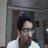Investment Banking Analyst at Kotak Investment Banking um Kotak Investment Banking
16. Apr 2013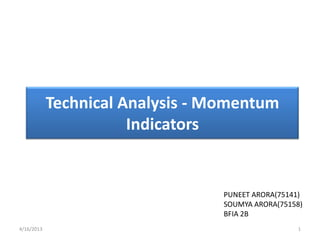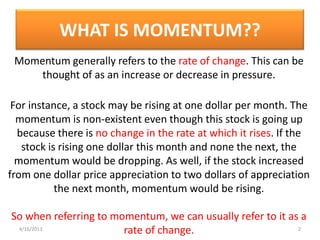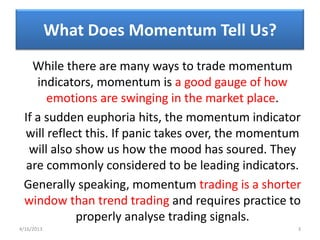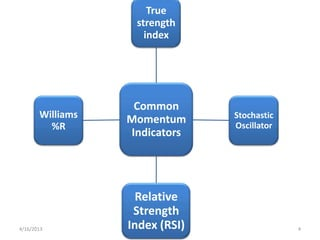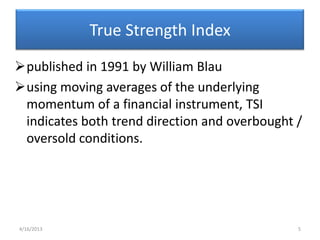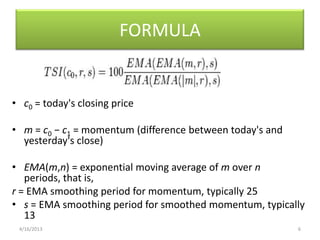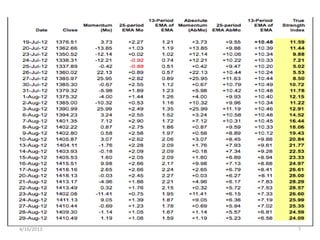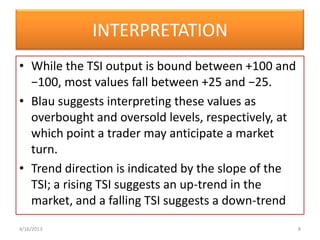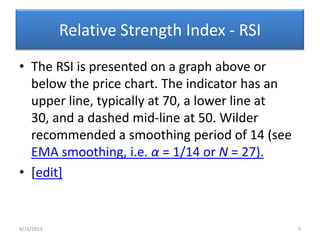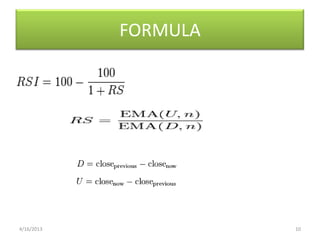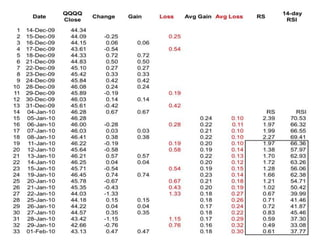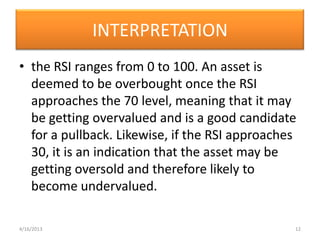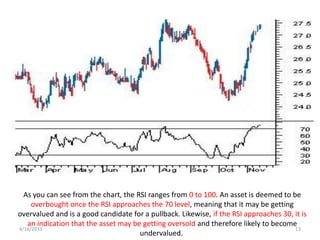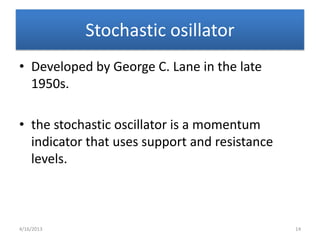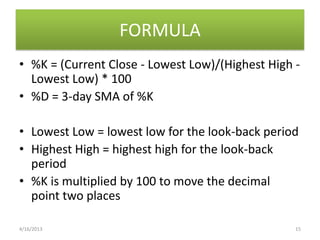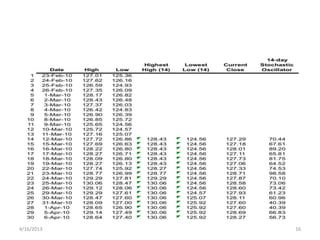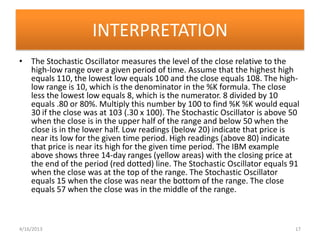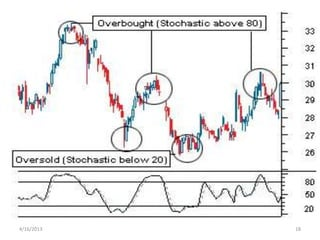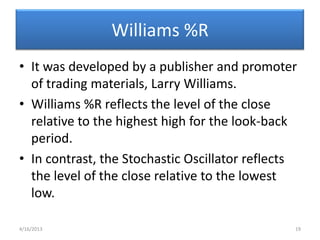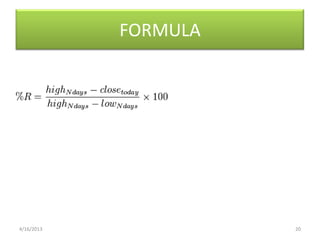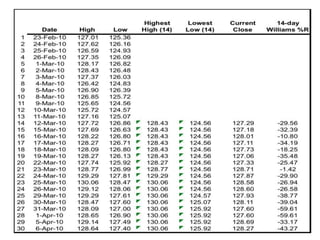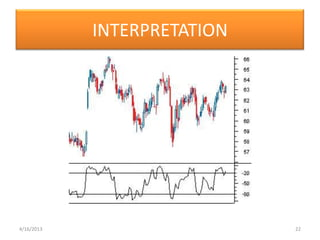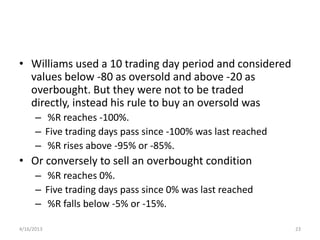1 von 24

### Momentum indicators in tech. analysis

• 1. Technical Analysis - Momentum Indicators PUNEET ARORA(75141) SOUMYA ARORA(75158) BFIA 2B 4/16/2013 1
• 2. WHAT IS MOMENTUM?? Momentum generally refers to the rate of change. This can be thought of as an increase or decrease in pressure. For instance, a stock may be rising at one dollar per month. The momentum is non-existent even though this stock is going up because there is no change in the rate at which it rises. If the stock is rising one dollar this month and none the next, the momentum would be dropping. As well, if the stock increased from one dollar price appreciation to two dollars of appreciation the next month, momentum would be rising. So when referring to momentum, we can usually refer to it as a 4/16/2013 rate of change. 2
• 3. What Does Momentum Tell Us? While there are many ways to trade momentum indicators, momentum is a good gauge of how emotions are swinging in the market place. If a sudden euphoria hits, the momentum indicator will reflect this. If panic takes over, the momentum will also show us how the mood has soured. They are commonly considered to be leading indicators. Generally speaking, momentum trading is a shorter window than trend trading and requires practice to properly analyse trading signals. 4/16/2013 3
• 4. True strength index Common Williams Momentum Stochastic %R Oscillator Indicators Relative Strength 4/16/2013 Index (RSI) 4
• 5. True Strength Index published in 1991 by William Blau using moving averages of the underlying momentum of a financial instrument, TSI indicates both trend direction and overbought / oversold conditions. 4/16/2013 5
• 6. FORMULA • c0 = today's closing price • m = c0 − c1 = momentum (difference between today's and yesterday's close) • EMA(m,n) = exponential moving average of m over n periods, that is, r = EMA smoothing period for momentum, typically 25 • s = EMA smoothing period for smoothed momentum, typically 13 4/16/2013 6
• 8. INTERPRETATION • While the TSI output is bound between +100 and −100, most values fall between +25 and −25. • Blau suggests interpreting these values as overbought and oversold levels, respectively, at which point a trader may anticipate a market turn. • Trend direction is indicated by the slope of the TSI; a rising TSI suggests an up-trend in the market, and a falling TSI suggests a down-trend 4/16/2013 8
• 9. Relative Strength Index - RSI • The RSI is presented on a graph above or below the price chart. The indicator has an upper line, typically at 70, a lower line at 30, and a dashed mid-line at 50. Wilder recommended a smoothing period of 14 (see EMA smoothing, i.e. α = 1/14 or N = 27). •  4/16/2013 9
• 11. 4/16/2013 11
• 12. INTERPRETATION • the RSI ranges from 0 to 100. An asset is deemed to be overbought once the RSI approaches the 70 level, meaning that it may be getting overvalued and is a good candidate for a pullback. Likewise, if the RSI approaches 30, it is an indication that the asset may be getting oversold and therefore likely to become undervalued. 4/16/2013 12
• 13. As you can see from the chart, the RSI ranges from 0 to 100. An asset is deemed to be overbought once the RSI approaches the 70 level, meaning that it may be getting overvalued and is a good candidate for a pullback. Likewise, if the RSI approaches 30, it is an indication that the asset may be getting oversold and therefore likely to become 4/16/2013 13 undervalued.
• 14. Stochastic osillator • Developed by George C. Lane in the late 1950s. • the stochastic oscillator is a momentum indicator that uses support and resistance levels. 4/16/2013 14
• 15. FORMULA • %K = (Current Close - Lowest Low)/(Highest High - Lowest Low) * 100 • %D = 3-day SMA of %K • Lowest Low = lowest low for the look-back period • Highest High = highest high for the look-back period • %K is multiplied by 100 to move the decimal point two places 4/16/2013 15
• 16. 4/16/2013 16
• 17. INTERPRETATION • The Stochastic Oscillator measures the level of the close relative to the high-low range over a given period of time. Assume that the highest high equals 110, the lowest low equals 100 and the close equals 108. The high- low range is 10, which is the denominator in the %K formula. The close less the lowest low equals 8, which is the numerator. 8 divided by 10 equals .80 or 80%. Multiply this number by 100 to find %K %K would equal 30 if the close was at 103 (.30 x 100). The Stochastic Oscillator is above 50 when the close is in the upper half of the range and below 50 when the close is in the lower half. Low readings (below 20) indicate that price is near its low for the given time period. High readings (above 80) indicate that price is near its high for the given time period. The IBM example above shows three 14-day ranges (yellow areas) with the closing price at the end of the period (red dotted) line. The Stochastic Oscillator equals 91 when the close was at the top of the range. The Stochastic Oscillator equals 15 when the close was near the bottom of the range. The close equals 57 when the close was in the middle of the range. 4/16/2013 17
• 18. 4/16/2013 18
• 19. Williams %R • It was developed by a publisher and promoter of trading materials, Larry Williams. • Williams %R reflects the level of the close relative to the highest high for the look-back period. • In contrast, the Stochastic Oscillator reflects the level of the close relative to the lowest low. 4/16/2013 19
• 21. 4/16/2013 21
• 23. • Williams used a 10 trading day period and considered values below -80 as oversold and above -20 as overbought. But they were not to be traded directly, instead his rule to buy an oversold was – %R reaches -100%. – Five trading days pass since -100% was last reached – %R rises above -95% or -85%. • Or conversely to sell an overbought condition – %R reaches 0%. – Five trading days pass since 0% was last reached – %R falls below -5% or -15%. 4/16/2013 23